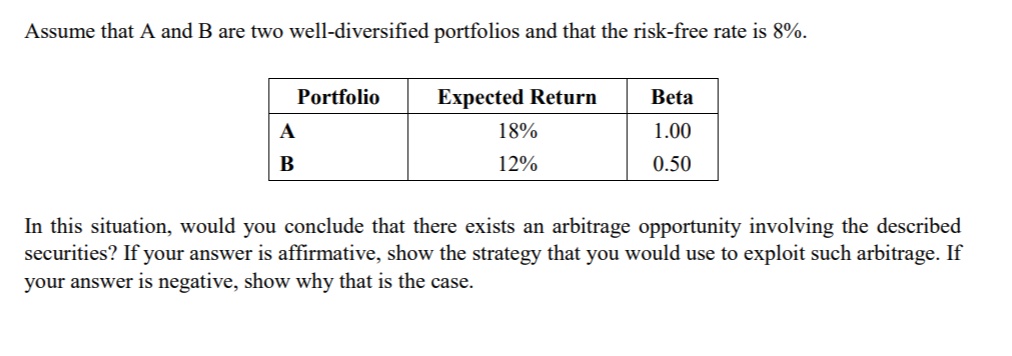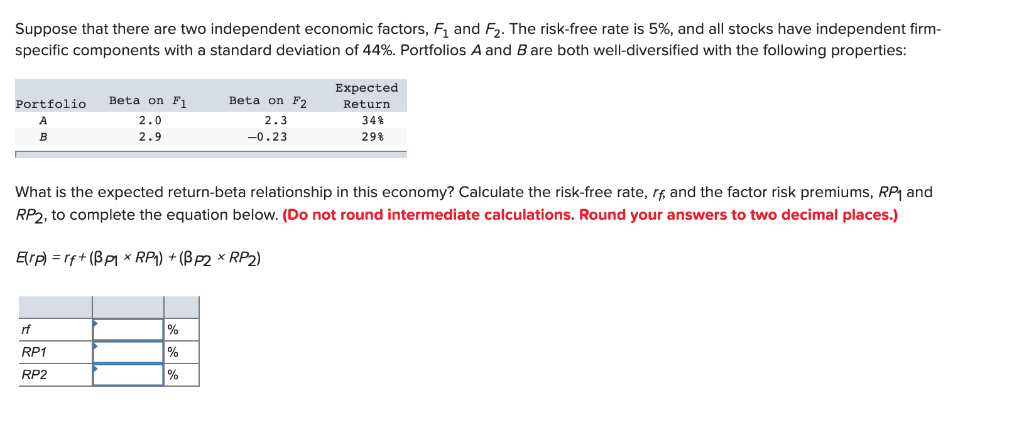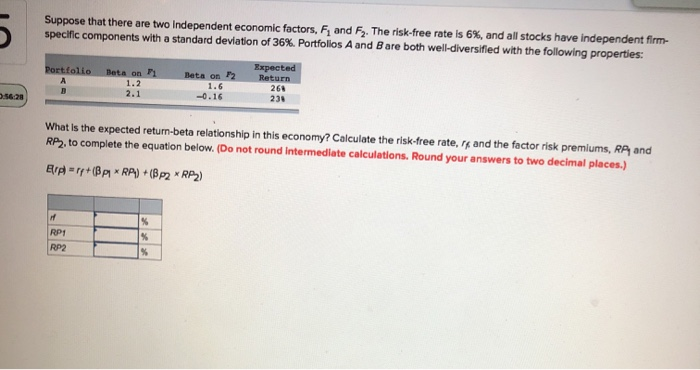# Please show all equations and work as needed. Assume that A and B are two well-diversified portfolios and that the risk-free rate is 8%. PortfolioExpected Returm 1.00 18% 12% 0.50 In this situation,...

Please show all equations and work as needed.Assume that A and B are two well-diversified portfolios and that the risk-free rate is 8%. PortfolioExpected Returm 1.00 18% 12% 0.50 In this situation, would you conclude that there exists an arbitrage opportunity involving the described securities? If your answer is affirmative, show the strategy that you would use to exploit such arbitrage. If your answer is negative, show why that is the case

According to A:

So, B's return should be=8%+0.5*10%=13%

But as B's return is 12%, so B is overvalued

Short sell B and buy/long A

##### Add Answer of: Please show all equations and work as needed. Assume that A and B are two well-diversified portfolios and that the risk-free rate is 8%. PortfolioExpected Returm 1.00 18% 12% 0.50 In this situation,...
Similar Homework Help Questions
• ### Suppose that there are two independent economic factors, F1 and F2. The risk-free rate is 5%, and...Suppose that there are two independent economic factors, F1 and F2. The risk-free rate is 5%, and all stocks have independent firm-specific components with a standard deviation of 44%. Portfolios A and B are both well-diversified with the following properties: Suppose that there are two independent economic factors, F1 and F2. The risk-free rate is 5%, and all stocks have independent firm- specific components with a standard deviation of 44%. Portfolios A and B are both well-diversified with the following...

• ### Suppose that there are two Independent economic factors, F and F. The risk-free rate is 6%, and all stocks have ind...Suppose that there are two Independent economic factors, F and F. The risk-free rate is 6%, and all stocks have independent firm specific components with a standard deviation of 36%. Portfolios A and B are both well-diversified with the following properties: Portfolio Deta on 2 Expected Return 1.2 Beta on -0.16 266 230 What is the expected return-beta relationship in this economy? Calculate the risk-free rate, and the factor risk premiums, RA and RP2, to complete the equation below. (Do...

• ### As an investor you are faced with two choices investing in a risky fund which has a return of 12% and a standard deviation of 20% and risk free asset which has a return of 6%

As an investor you are faced with two choices investing in a risky fund which has a return of 12% and a standard deviation of 20% and risk free asset which has a return of 6%. 1)As a risk averse investor, my objectives is to invest in a portfolio of a 15% risk.What would be the allocation between risk free and risk assets of my investment? 2)My brother is less risk averse than me, and he is trying to acheive...

Need Online Homework Help?